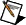# Optimizing Design Parameters (Control Design and Simulation Module)

One important application of simulating dynamic system models is using the simulation to determine parameter values that maximize some measure of performance. The LabVIEW Control Design and Simulation Module includes the SIM Optimal Design VI, which you can use to obtain parameters that minimize a cost function while satisfying constraints on a dynamic system. You can use this VI with both linear and nonlinear systems, although the Control Design and Simulation Module includes pre-defined options for only linear systems.

Design problems can range from designing physical elements, such as springs, to designing more abstract elements such as controllers or digital filters. Correspondingly, performance specifications might range from simple mechanical limits on outputs to more sophisticated requirements such as frequency domain norms for controlled systems.

For example, when designing a suspension system for a car, you must select a stiffness constant for a spring and a damping constant for a dissipative element. The goal is to find a parameter set that provides maximum comfort. This optimal parameter set corresponds to a performance measure, such as the average deviation of the passenger from a desired height as the car travels down the road. You use parameter design to determine this optimal parameter set while taking into account the dynamics of the system and the expected operating conditions and disturbances.

You can use several techniques to determine this parameter set. For some problems, you might be able to compute the optimum analytically. However, analytical solutions typically are difficult or impossible to compute. In such cases, you can use numerical optimization instead. A powerful and general purpose numerical optimization algorithm is Sequential Quadratic Programming (SQP). The SIM Optimal Design VI uses this algorithm. This VI provides domain-specific functions you can use to perform parameter optimization for design purposes. Specifically, you can use this VI to determine optimal parameters from finite-horizon time-domain dynamics simulations.

The following expressions define the nonlinear optimization problem.

min(J(p))

hlH(p) ≤ hu

plppu

where p is a parameter value, J(p) is a cost function, and H(p) is a set of constraints. The objective of the SQP algorithm is to minimize J(p) and satisfy the constraints defined by hlH(p) ≤ hu while keeping p within specified minimum and maximum values.

Designing a system using the SQP algorithm involves the following steps:

1. Constructing the dynamic system model and specifying the component of that model for which you want to find optimal parameter values.
2. Defining a performance measure, also known as a cost function, you want to minimize.
3. Defining any constraints on the dynamic system that any feasible parameter values must satisfy.
4. Defining minimum and maximum values for each parameter.
5. Defining a set of initial parameter values and an initial parameters mesh, which generates additional sets of initial parameter values.
6. Executing the SQP algorithm, using the information you specified in steps 1 through 5, by running the SIM Optimal Design VI.

The cost function, inequality constraints, and component to optimize make up the Problem Specification parameter of the SIM Optimal Design VI. For each option, you can choose from pre-defined types or specify a customized version.Note  The Control Design and Simulation Module includes a case study that determines the optimal parameters for a proportional-integral-derivative (PID) controller.